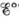# Calculate values for the following cells. Which reactions are spontaneous as written (under standard conditions)? Balance the equations that are not already balanced. Standard reduction potentials are found in Table 17-1. a. H 2 ( g ) → H + ( a q ) + H − ( a q ) b. Au 3 + ( a q ) + Ag ( s ) → Ag + ( a q ) + Au ( s )### Chemistry: An Atoms First Approach

2nd Edition
Steven S. Zumdahl + 1 other
Publisher: Cengage Learning
ISBN: 9781305079243

#### Solutions

Chapter
Section### Chemistry: An Atoms First Approach

2nd Edition
Steven S. Zumdahl + 1 other
Publisher: Cengage Learning
ISBN: 9781305079243
Chapter 17, Problem 48E
Textbook Problem
22 views

## Calculatevalues for the following cells. Which reactions are spontaneous as written (under standard conditions)? Balance the equations that are not already balanced. Standard reduction potentials are found in Table 17-1.a. H 2 ( g ) → H + ( a q ) + H − ( a q ) b. Au 3 + ( a q ) + Ag ( s ) → Ag + ( a q ) + Au ( s )

(a)

Interpretation Introduction

Interpretation:

The reactions taking place in two different galvanic cells are given. The E° of the given galvanic cells, the spontaneity of the reactions and balanced chemical equation for each cell is to be stated.

Concept introduction:

The galvanic cell converts chemical energy into electrical energy while the electrolytic cell converts electrical energy into chemical energy.

The species at anode undergoes oxidation while the species at cathode undergoes reduction and the electrons generated at the anode are transferred through wire to the cathode.

To determine: The set up of the cell given in the chemical equation and the direction of electron flow, identification of the cathode and anode and balanced chemical equation.

### Explanation of Solution

The value of E°cell is calculated as -2.23V_ .

The reaction taking place at cathode is,

H2(g)+2e2H(aq)E°red=2.23V

The reaction taking place at anode is,

H2(g)2H+(aq)+2eE°ox=0.00V

Add both the oxidation and reduction half-reaction,

H2(g)+2e2H(aq)H2(g)2H+(aq)+2e

The final equation is,

H2(g)H(aq)+H+(aq)

The overall cell potential is calculated as,

E°cell=E°ox+E°red=0

(b)

Interpretation Introduction

Interpretation:

The reactions taking place in two different galvanic cells are given. The E° of the given galvanic cells, the spontaneity of the reactions and balanced chemical equation for each cell is to be stated.

Concept introduction:

The galvanic cell converts chemical energy into electrical energy while the electrolytic cell converts electrical energy into chemical energy.

The species at anode undergoes oxidation while the species at cathode undergoes reduction and the electrons generated at the anode are transferred through wire to the cathode.

To determine: The set up of the cell given in the chemical equation and the direction of electron flow, identification of the cathode and anode and the balanced chemical equation.

### Still sussing out bartleby?

Check out a sample textbook solution.

See a sample solution

#### The Solution to Your Study Problems

Bartleby provides explanations to thousands of textbook problems written by our experts, many with advanced degrees!

Get Started

Find more solutions based on key concepts
In high doses, tryptophan can improve nausea and skin disorders. T F

Nutrition: Concepts and Controversies - Standalone book (MindTap Course List)

Is ATP involved in anabolic processes or catabolic processes?

Chemistry for Today: General, Organic, and Biochemistry

What is physically exchanged during crossing over?

Human Heredity: Principles and Issues (MindTap Course List)

A hydrogen atom has a diameter of 1.06 X 10-10 m. The nucleous of the hydrogen atom has a diameter of approxima...

Physics for Scientists and Engineers, Technology Update (No access codes included)